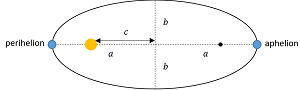# A newly discovered planet orbits a star with the same mass as the Sun with a semi-major axis of...

## Question:

A newly discovered planet orbits a star with the same mass as the Sun with a semi-major axis of 239.36 million kilometers. Its orbital eccentricity is 0.1.

A. Find the planet's orbital period.

B. Find the planet's perihelion distance.

C. Find the planet's aphelion distance.

## Ellipse

Ellipses are the geometrical place of the points in the plane such that the sum of their distance to two fixed points (focal points) is a constant. Mathematically, the ellipse is described by the equation,

{eq}\dfrac{x^2}{a^2}+\dfrac{y^2}{b^2}=1 {/eq},

where {eq}a {/eq} and {eq}b {/eq} are the semi-axes of the ellipse. {eq}a {/eq} is the major semi-axis and {eq}b {/eq} the minor semi-axis. The above equation represents a horizontal ellipse since the major semi-axis corresponds to {eq}x {/eq}.

The ellipse eccentricity is defined as the ratio between half the focal distance and the major semi-axis,

{eq}\varepsilon=\dfrac{c}{a} {/eq}.

The two semi-axes are related by the equation,

{eq}a^2=b^2+c^2 {/eq}.

A. Using Kepler's third law we can determine the orbital period of the planet,

{eq}T=\dfrac{2\pi a^{3/2}}{\sqrt{G M}} {/eq},

where {eq}G=6.67 \times 10^{-11} \;\rm N\cdot m^2/kg^2 {/eq} is the universal gravitational constant, {eq}a=239.36 \times 10^9 \;\rm m {/eq} is the semi-major axis of the elliptical orbit and {eq}M=1.99\times 10^30 \;\rm kg {/eq}.

Evaluating the expression,

{eq}T=\dfrac{2 \cdot 3.1416 \cdot (239.36 \times 10^9 \;\rm m)^{3/2}}{\sqrt{6.67 \times 10^{-11} \;\rm N\cdot m^2/kg^2 \cdot 1.99\times 10^30 \;\rm kg}}=\boxed{6.39 \times 10^7 \;\rm s} {/eq}.

The period of the planet orbiting the star is {eq}2.03 \;\rm years {/eq}.

B. The perihelion distance is the shortest possible distance between the planet and the star. The diagram below shows the schematic for the perihelion and the aphelion.From the diagram, we can see that the perihelion distance is,

{eq}per=a-c {/eq},

the difference between the semi-major axis length and half the focal distance.

To determine {eq}c {/eq} we employ the information about the orbit's eccentricity,

{eq}c=\varepsilon a=0.1 \cdot 239.36 \times 10^9 \;\rm m=239.36 \times 10^8 \;\rm m {/eq}.

Consequently, the perihelion distance is,

{eq}per=239.36 \times 10^9 \;\rm m-239.36 \times 10^8 \;\rm m=\boxed{215.42 \times 10^9 \;\rm m} {/eq}.

C. Proceeding in the same fashion for the aphelion,

{eq}aph=a+c {/eq},

the sum between the semi-major axis length and half the focal distance.

{eq}aph=239.36 \times 10^9 \;\rm m+239.36 \times 10^8 \;\rm m=\boxed{263.30 \times 10^9 \;\rm m} {/eq}.